# support vector machine 1.pptx

13 de Sep de 2022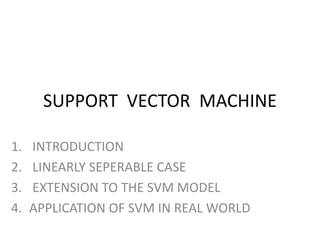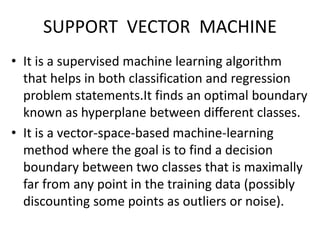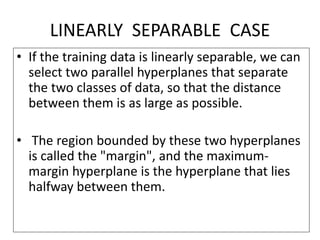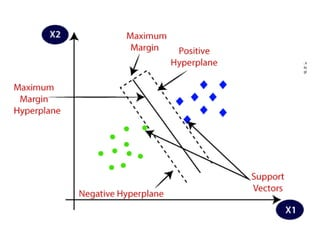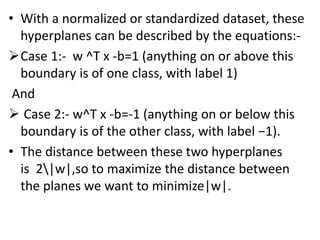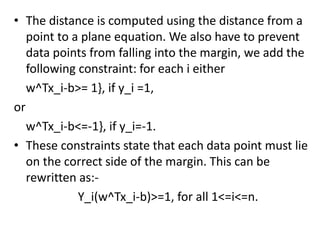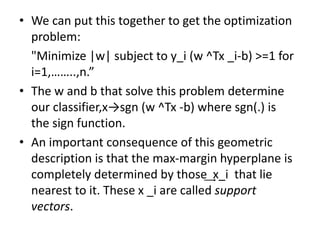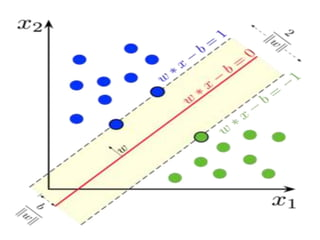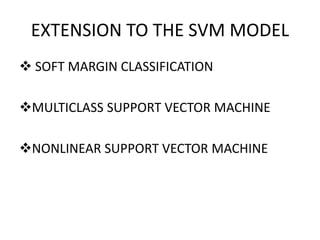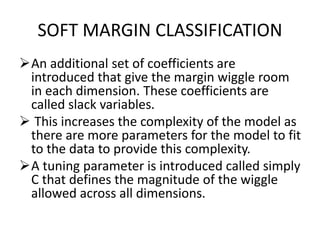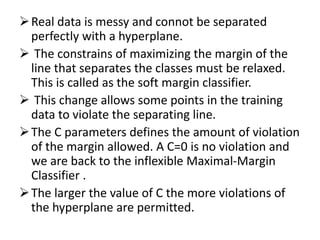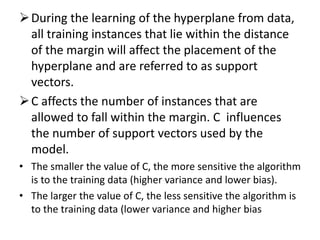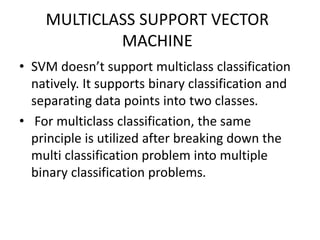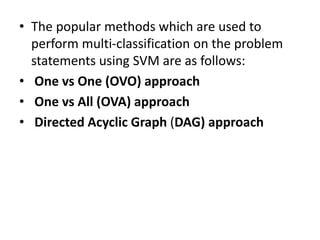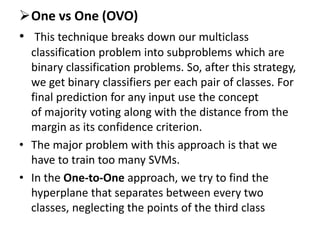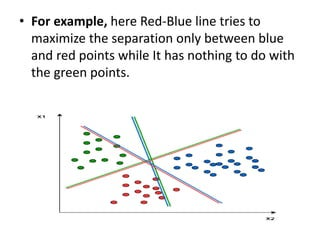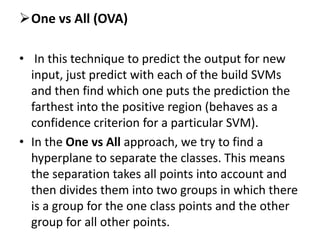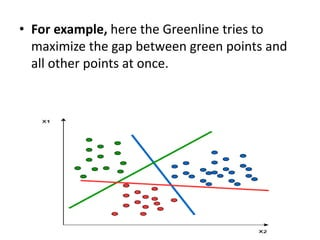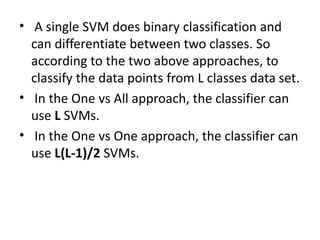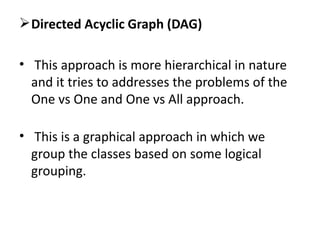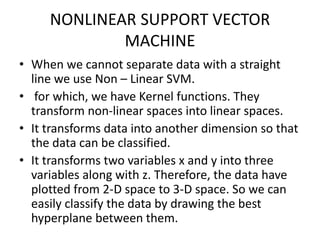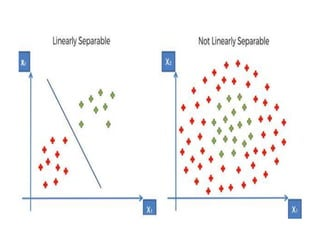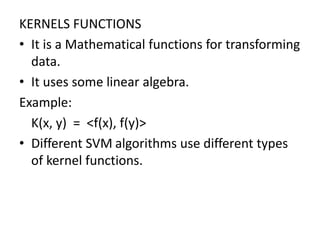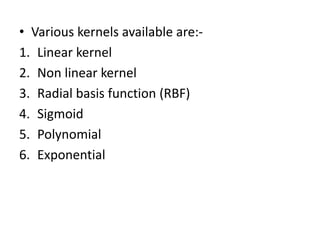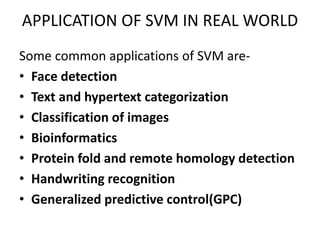1 de 25

### support vector machine 1.pptx

• 1. SUPPORT VECTOR MACHINE 1. INTRODUCTION 2. LINEARLY SEPERABLE CASE 3. EXTENSION TO THE SVM MODEL 4. APPLICATION OF SVM IN REAL WORLD
• 2. SUPPORT VECTOR MACHINE • It is a supervised machine learning algorithm that helps in both classification and regression problem statements.It finds an optimal boundary known as hyperplane between different classes. • It is a vector-space-based machine-learning method where the goal is to find a decision boundary between two classes that is maximally far from any point in the training data (possibly discounting some points as outliers or noise).
• 3. LINEARLY SEPARABLE CASE • If the training data is linearly separable, we can select two parallel hyperplanes that separate the two classes of data, so that the distance between them is as large as possible. • The region bounded by these two hyperplanes is called the "margin", and the maximum- margin hyperplane is the hyperplane that lies halfway between them.
• 5. • With a normalized or standardized dataset, these hyperplanes can be described by the equations:- Case 1:- w ^T x -b=1 (anything on or above this boundary is of one class, with label 1) And  Case 2:- w^T x -b=-1 (anything on or below this boundary is of the other class, with label −1). • The distance between these two hyperplanes is 2|w|,so to maximize the distance between the planes we want to minimize|w|.
• 6. • The distance is computed using the distance from a point to a plane equation. We also have to prevent data points from falling into the margin, we add the following constraint: for each i either w^Tx_i-b>= 1}, if y_i =1, or w^Tx_i-b<=-1}, if y_i=-1. • These constraints state that each data point must lie on the correct side of the margin. This can be rewritten as:- Y_i(w^Tx_i-b)>=1, for all 1<=i<=n.
• 7. • We can put this together to get the optimization problem: "Minimize |w| subject to y_i (w ^Tx _i-b) >=1 for i=1,……..,n.” • The w and b that solve this problem determine our classifier,x→sgn (w ^Tx -b) where sgn(.) is the sign function. • An important consequence of this geometric description is that the max-margin hyperplane is completely determined by those ͢ x_i that lie nearest to it. These x _i are called support vectors.
• 9. EXTENSION TO THE SVM MODEL  SOFT MARGIN CLASSIFICATION MULTICLASS SUPPORT VECTOR MACHINE NONLINEAR SUPPORT VECTOR MACHINE
• 10. SOFT MARGIN CLASSIFICATION An additional set of coefficients are introduced that give the margin wiggle room in each dimension. These coefficients are called slack variables.  This increases the complexity of the model as there are more parameters for the model to fit to the data to provide this complexity. A tuning parameter is introduced called simply C that defines the magnitude of the wiggle allowed across all dimensions.
• 11. Real data is messy and connot be separated perfectly with a hyperplane.  The constrains of maximizing the margin of the line that separates the classes must be relaxed. This is called as the soft margin classifier.  This change allows some points in the training data to violate the separating line. The C parameters defines the amount of violation of the margin allowed. A C=0 is no violation and we are back to the inflexible Maximal-Margin Classifier . The larger the value of C the more violations of the hyperplane are permitted.
• 12. During the learning of the hyperplane from data, all training instances that lie within the distance of the margin will affect the placement of the hyperplane and are referred to as support vectors. C affects the number of instances that are allowed to fall within the margin. C influences the number of support vectors used by the model. • The smaller the value of C, the more sensitive the algorithm is to the training data (higher variance and lower bias). • The larger the value of C, the less sensitive the algorithm is to the training data (lower variance and higher bias
• 13. MULTICLASS SUPPORT VECTOR MACHINE • SVM doesn’t support multiclass classification natively. It supports binary classification and separating data points into two classes. • For multiclass classification, the same principle is utilized after breaking down the multi classification problem into multiple binary classification problems.
• 14. • The popular methods which are used to perform multi-classification on the problem statements using SVM are as follows: • One vs One (OVO) approach • One vs All (OVA) approach • Directed Acyclic Graph (DAG) approach
• 15. One vs One (OVO) • This technique breaks down our multiclass classification problem into subproblems which are binary classification problems. So, after this strategy, we get binary classifiers per each pair of classes. For final prediction for any input use the concept of majority voting along with the distance from the margin as its confidence criterion. • The major problem with this approach is that we have to train too many SVMs. • In the One-to-One approach, we try to find the hyperplane that separates between every two classes, neglecting the points of the third class
• 16. • For example, here Red-Blue line tries to maximize the separation only between blue and red points while It has nothing to do with the green points.
• 17. One vs All (OVA) • In this technique to predict the output for new input, just predict with each of the build SVMs and then find which one puts the prediction the farthest into the positive region (behaves as a confidence criterion for a particular SVM). • In the One vs All approach, we try to find a hyperplane to separate the classes. This means the separation takes all points into account and then divides them into two groups in which there is a group for the one class points and the other group for all other points.
• 18. • For example, here the Greenline tries to maximize the gap between green points and all other points at once.
• 19. • A single SVM does binary classification and can differentiate between two classes. So according to the two above approaches, to classify the data points from L classes data set. • In the One vs All approach, the classifier can use L SVMs. • In the One vs One approach, the classifier can use L(L-1)/2 SVMs.
• 20. Directed Acyclic Graph (DAG) • This approach is more hierarchical in nature and it tries to addresses the problems of the One vs One and One vs All approach. • This is a graphical approach in which we group the classes based on some logical grouping.
• 21. NONLINEAR SUPPORT VECTOR MACHINE • When we cannot separate data with a straight line we use Non – Linear SVM. • for which, we have Kernel functions. They transform non-linear spaces into linear spaces. • It transforms data into another dimension so that the data can be classified. • It transforms two variables x and y into three variables along with z. Therefore, the data have plotted from 2-D space to 3-D space. So we can easily classify the data by drawing the best hyperplane between them.
• 23. KERNELS FUNCTIONS • It is a Mathematical functions for transforming data. • It uses some linear algebra. Example: K(x, y) = <f(x), f(y)> • Different SVM algorithms use different types of kernel functions.
• 24. • Various kernels available are:- 1. Linear kernel 2. Non linear kernel 3. Radial basis function (RBF) 4. Sigmoid 5. Polynomial 6. Exponential
• 25. APPLICATION OF SVM IN REAL WORLD Some common applications of SVM are- • Face detection • Text and hypertext categorization • Classification of images • Bioinformatics • Protein fold and remote homology detection • Handwriting recognition • Generalized predictive control(GPC)